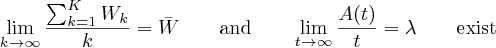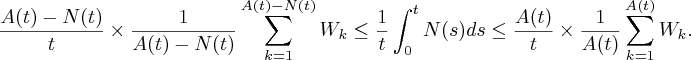# Little’s LawHere$\bar{N}$ is the long run queue length;$\bar{W}$ is the expected waiting time;$\lambda$ is the arrival rate at the queue.

Little’s Law is one of applied probability’s most robust results.

•$N(t)$ is the number of customers in a queue at time$t\geq 0$.
•$A(t)$ is the total number of arrivals at the queue by time$t\geq 0$.$A(0)$ includes customers at the queue at time$0$.
• Let$W_k$ be the waiting time of the$k$-th arrival at the queue. That is the time from arrival until departure.

It relates mean queue length to waiting times and arrival rates.

Lemma (Little’s Law): We assume the number of customers$N(t)$ is smaller than$t$:Assuming there is a long run waiting time$\bar{W}$ and a long run arrival rate$\lambda$, that is there are well defined limits:then the long run queue length$\bar{N}=\lim_{t\rightarrow\infty} \frac{1}{t} \int_0^t N(s)ds$ is well defined and is given by Little’s Law:Proof: The average number of customers in the queue bounded above by sum of the waiting times of all arriving customers and is lower bounded by the sum of waiting times of the customers who have completed service. I.e.ThusLetting$t\rightarrow\infty$, we gain$\lambda \times \bar{W} \leq \bar{N} \leq \lambda \times \bar{W}$, as required.$\square$